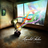首页   注册   登录
V2EX = way to explore
V2EX 是一个关于分享和探索的地方

V2EX  ›  分享创造

# AnyID - 万能的 ID 生成器

aleung · 2017-02-23 13:52:16 +08:00 · 2113 次点击

AnyID 生成的 ID 为字符串（也可以纯数字），信息密度尽可能的高，也就是用最少的位数表示尽量多的信息。

AnyID 设计的首要考虑原则是 API 的直观易用。看看这些例子：

#### 指定长度，随机值填充

21 个字符，包含大写小写字母和数字。 这个 ID 的碰撞可能性跟 type 4 (random) UUID 一样低。

``````const ids = anyid().encode('Aa0').length(21).random()
const id  = ids.id();
``````
``````1LrKcmd0uk1Ma8szUxtda
``````

#### 多个分段

``````const ids = anyid()
.encode('0A-IO')
.section( anyid().fixed(process.pid) )
.delimiter('-')
.section( anyid().time() );
``````

``````008CL-00TYMZS0P3
``````

#### 自增序列，指定位(bit)长度

``````const ids = anyid()
.encode('0')
.bit(41).time().since(new Date('2016-7-1'))
.bit(12).seq().resetByTime();
.bit(10).fix(workerId);
``````

``````071243223959339218
``````

#### 函数值

``````const nanotime = () => {
return process.hrtime();
};

const ids = anyid()
.encode('Aa0')
.section( anyid().time('s') )
.delimiter('+')
.section( anyid().of(nanotime) );
``````
``````BlX6bX+j3Uz0
``````

#### 参数绑定

``````const ids = anyid()
.encode('Aa0')
.section( anyid().variable('countryId') )
.delimiter('-')
.section( anyid().variable('userId') )
.delimiter('-')
.section( anyid().length(5).random() );

const id = ids.id({ countryId: 86, userId: 635023 });
``````
``````AAABY-ACpMT-EBwQJ
``````
1 回复  |  直到 2017-02-24 10:42:06 +08:001 Wichna   2017-02-24 10:42:06 +08:00 非常赞👍